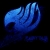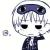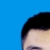## mysql如何计算两个表的值，插入另外一张表中

1. 原始表：
table1 （A，B，C，D）

 table1 A B C D A01 B01 1 3 A01 B01 2 4 A02 B02 3 1 A02 B02 2 3 A03 B03 1 3

table2（A，B，E，F）

 table2 A B E F A01 B01 1 3 A01 B01 6 4 A02 B02 3 8 A02 B02 2 3 A03 B03 6 9

2. 目标表：
table3（A，B，G，H，I，J）

 table3 A B G H I J A01 B01 2 4 6 4 A02 B02 3 3 3 8 A03 B03 1 3 6 9

3. 说明：
G=max(C)
H=max(D)
I=max(E)
J=max(F)

0insert into table3

select a.A,a.B,max(C),max(D),max(E),max(F) from table1 a,table2 b

where a.A=b.A and a.B=b.B group by a.A,a.B0http://blog.csdn.net/qq_25551295/article/details/47951197

0#### 引用来自“风翔飞”的评论

insert into table3

select a.A,a.B,max(C),max(D),max(E),max(F) from table1 a,table2 b

where a.A=b.A and a.B=b.B group by a.A,a.B